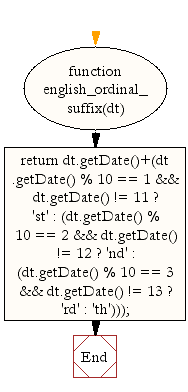# JavaScript: Get English ordinal suffix for the day of the month

## JavaScript Datetime: Exercise-23 with Solution

Write a JavaScript function to get English ordinal suffix for the day of the month, 2 characters (st, nd, rd or th.).

Test Data :
dt= new Date();
console.log(english_ordinal_suffix(dt));
"23rd"
dt = new Date(2015, 10, 1);
console.log(english_ordinal_suffix(dt));
"1st"

Sample Solution:-

HTML Code:

``````<!DOCTYPE html>
<html>
<head>
<meta charset="utf-8">
<title>JavaScript function to get English ordinal suffix for the day of the month, 2 characters (st, nd, rd or th.)</title>
</head>
<body>

</body>
</html>
```
```

JavaScript Code:

``````function english_ordinal_suffix(dt)
{
return dt.getDate()+(dt.getDate() % 10 == 1 && dt.getDate() != 11 ? 'st' : (dt.getDate() % 10 == 2 && dt.getDate() != 12 ? 'nd' : (dt.getDate() % 10 == 3 && dt.getDate() != 13 ? 'rd' : 'th')));
}

dt = new Date();
console.log(english_ordinal_suffix(dt));

dt = new Date(2015, 10, 1);
console.log(english_ordinal_suffix(dt));
```
```

Sample Output:

```20th
1st
```

Flowchart:Live Demo:

See the Pen JavaScript - Get English ordinal suffix for the day of the month-date-ex-23 by w3resource (@w3resource) on CodePen.

Improve this sample solution and post your code through Disqus

What is the difficulty level of this exercise?

﻿

## JavaScript: Tips of the Day

Convert tabs to spaces, where each tab corresponds to count spaces

Example:

```const tips_expandTabs = (str, count) => str.replace(/\t/g, ' '.repeat(count));
console.log(tips_expandTabs('\t\tw3resource', 4));
```

Output:

```"        w3resource"
```